ICSE Class 10 Maths Sample Question Paper – Mock 3 with Solutions can be accessed at BYJU’S, where both online and pdf can be downloaded. Detailed explanations of all sample papers for class 10 maths will help the students in revising their results. Hence, students can get all these solutions at BYJU’S that are given by our expert faculty.

Students who are preparing for the Class 10 Maths ICSE exam can score maximum marks by practising more questions on each concept. They can get ICSE Mock Sample Paper 3 here, which covers the entire syllabus and is prepared as per the board guidelines, with respect to the weightage for different concepts.

#### ICSE Class 10 Maths Mock Sample Paper 3 with Solutions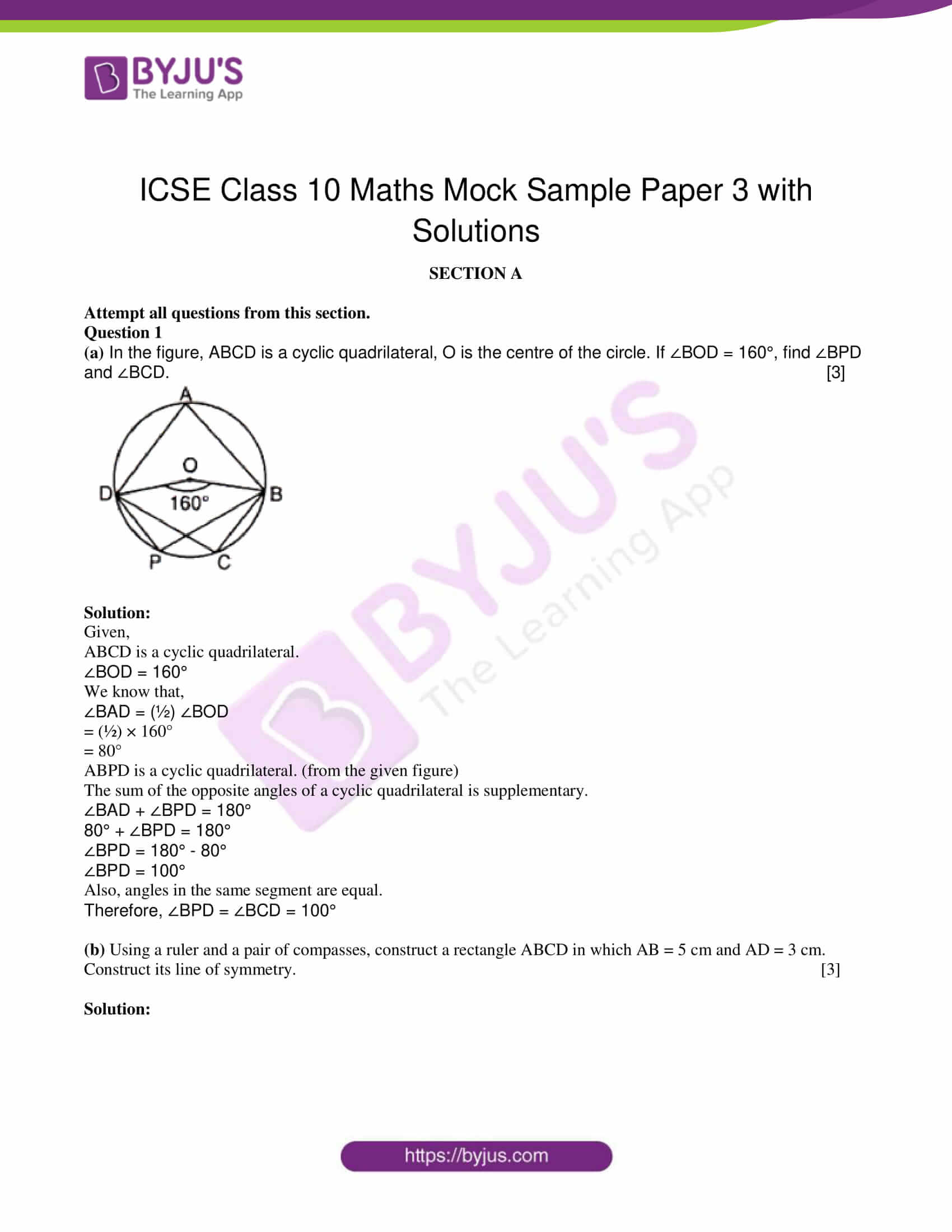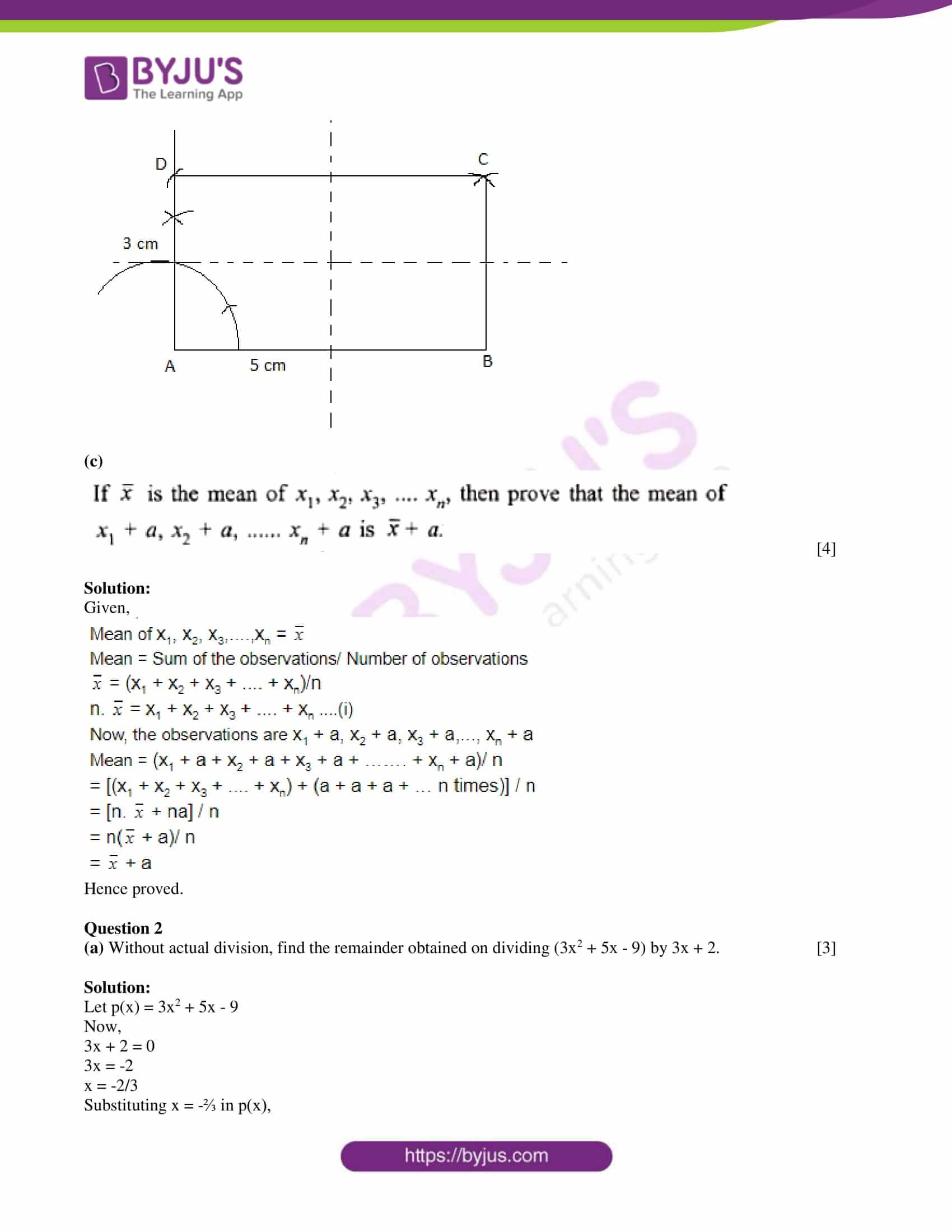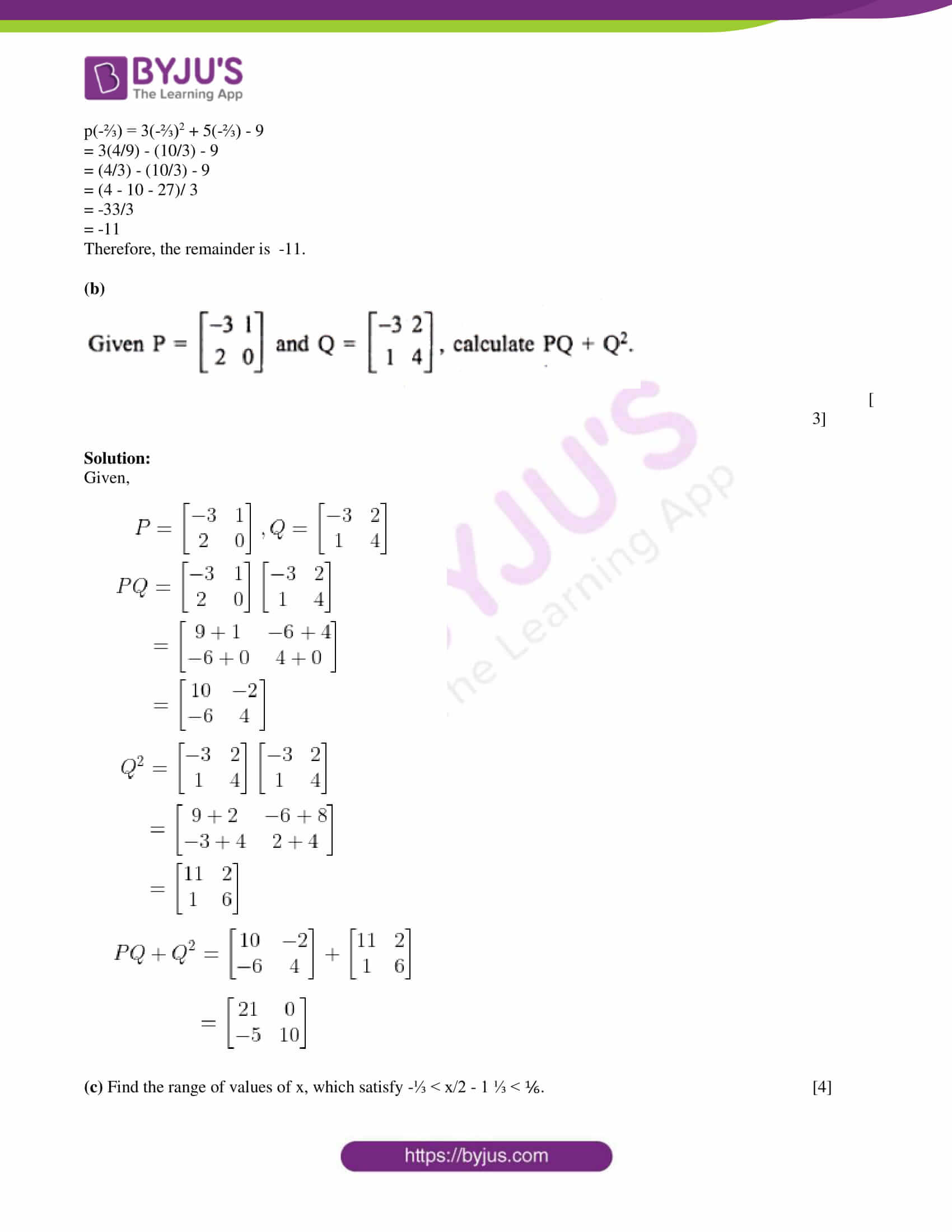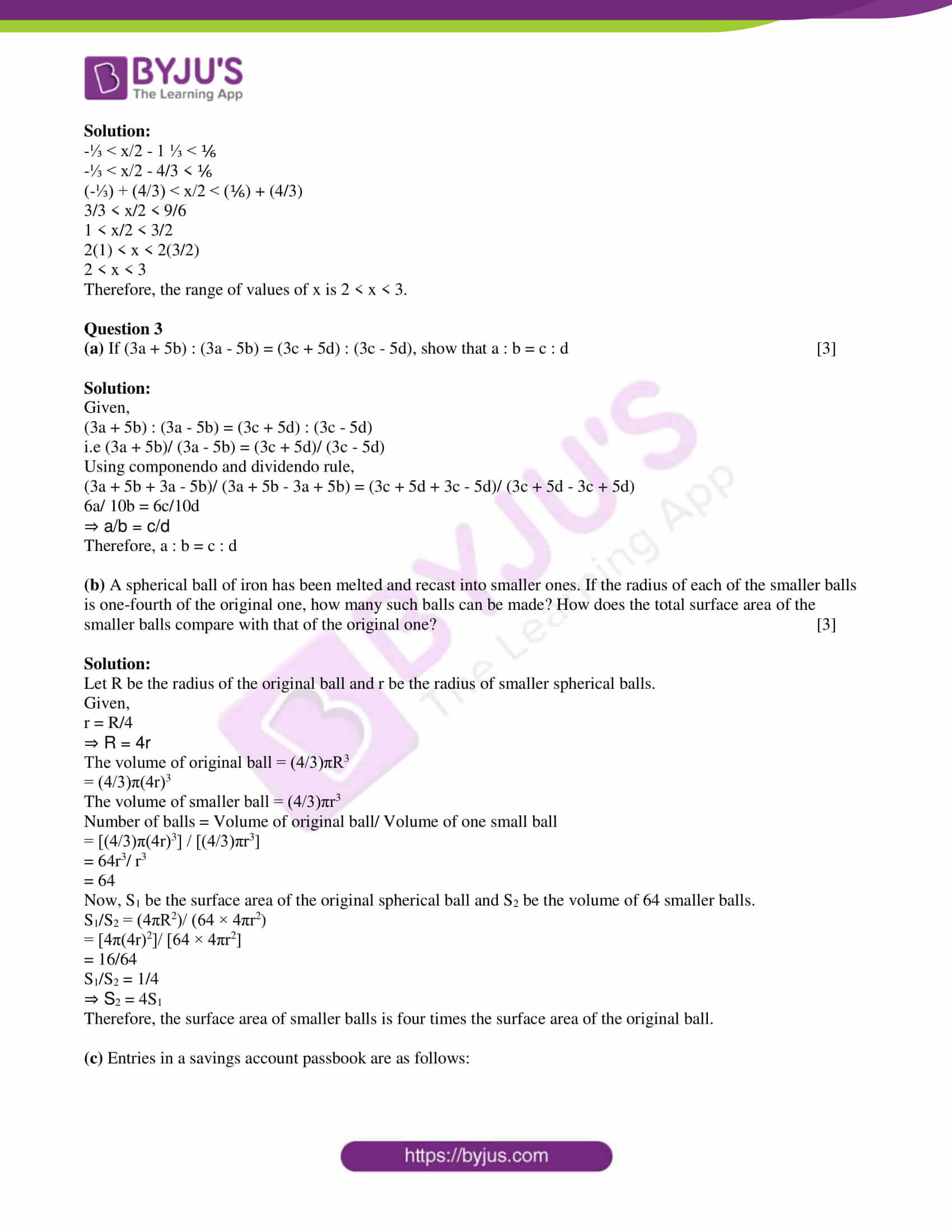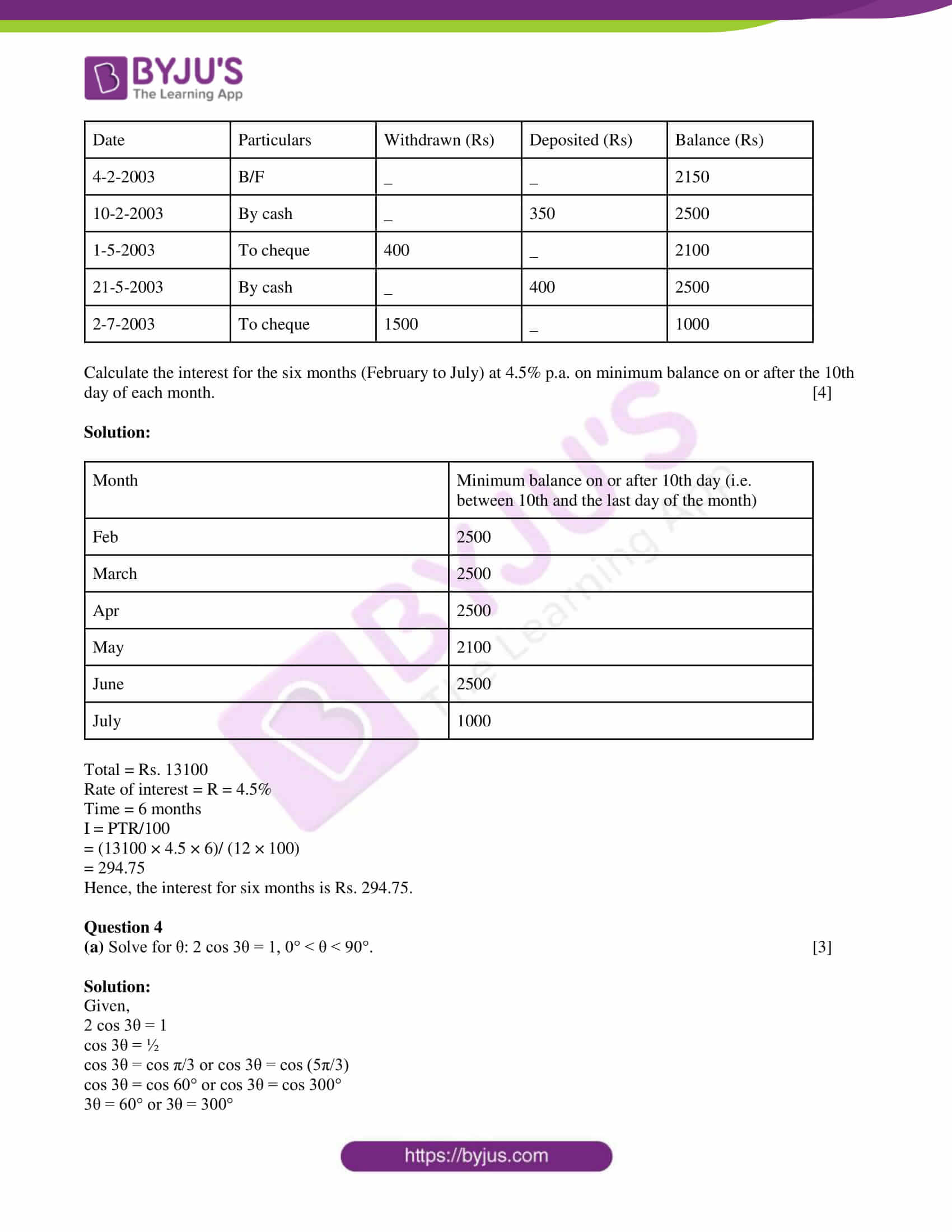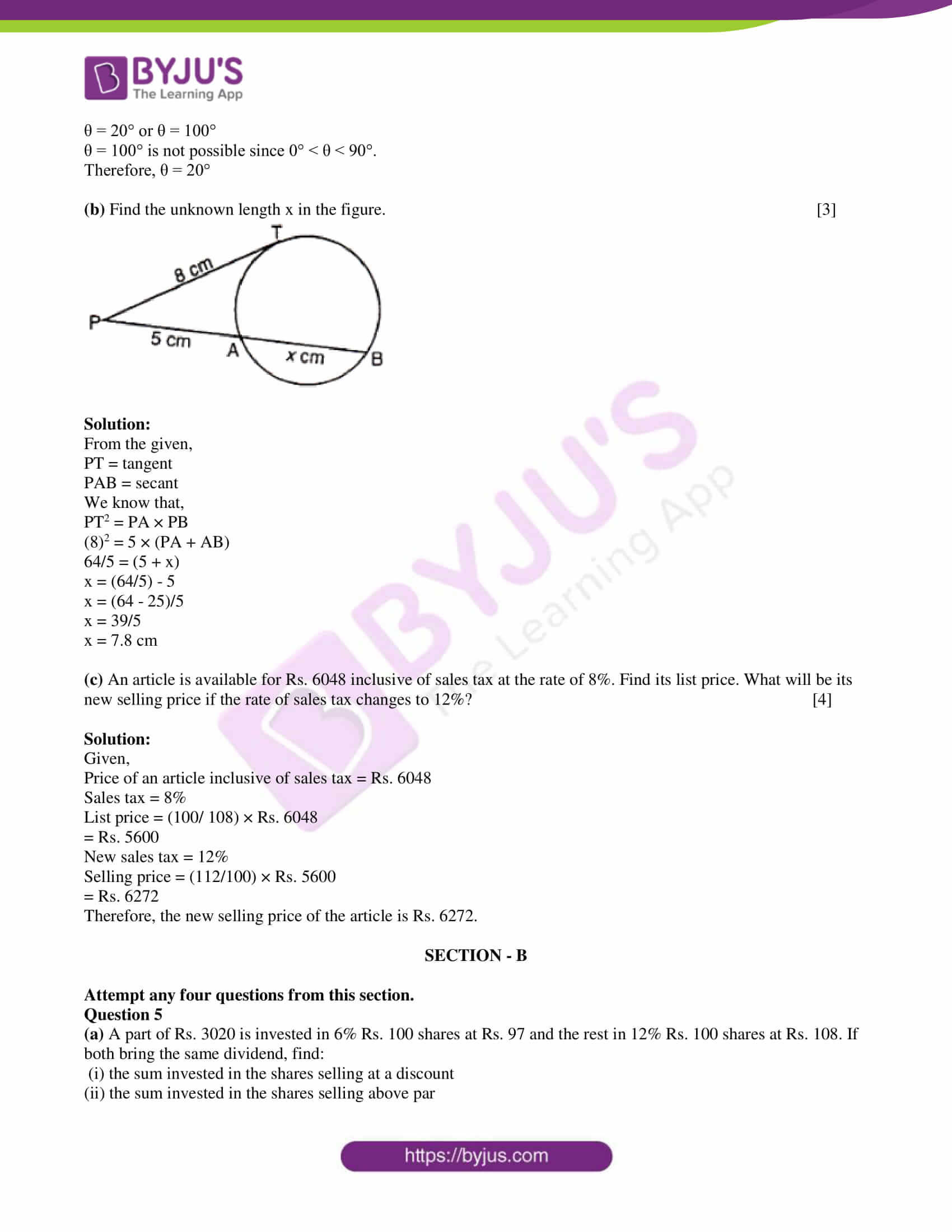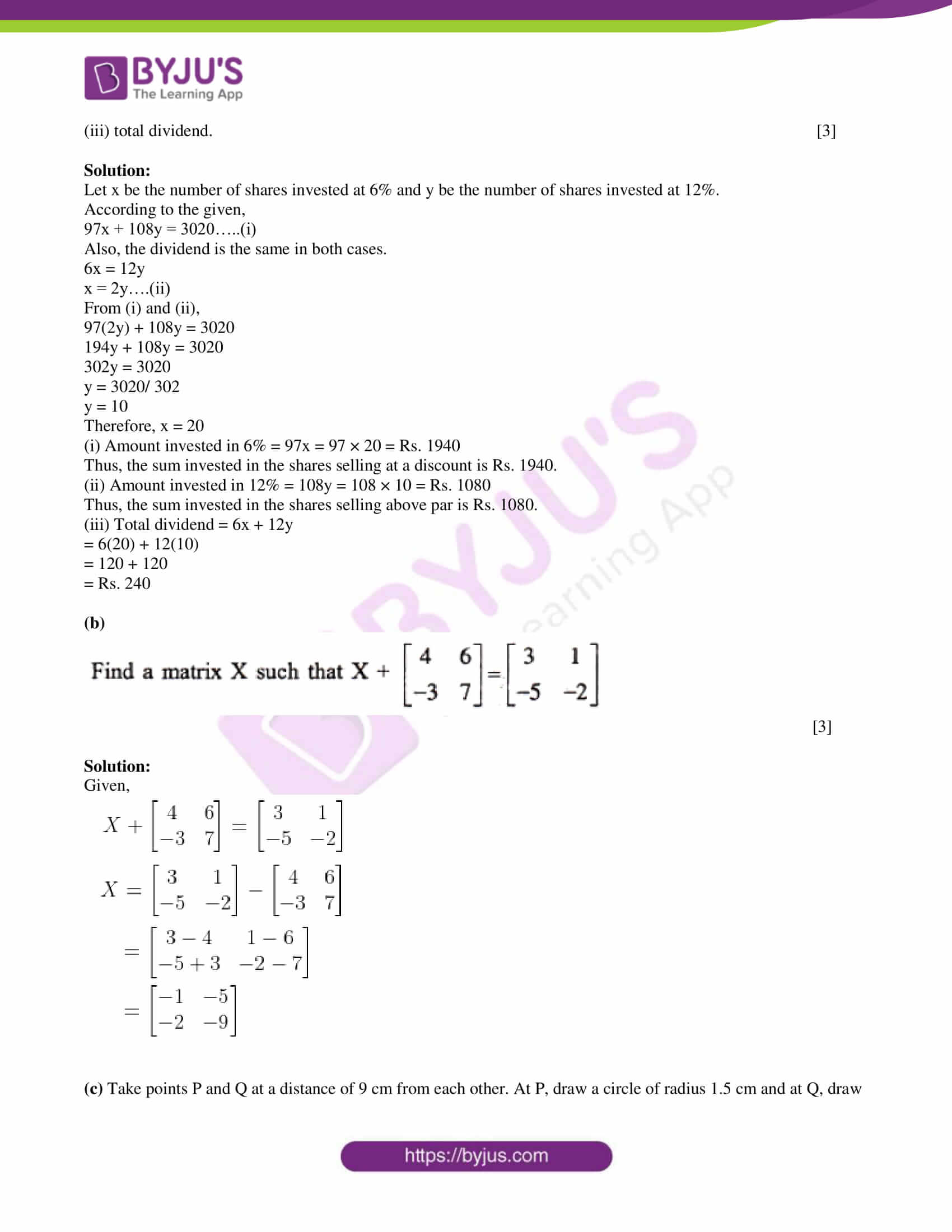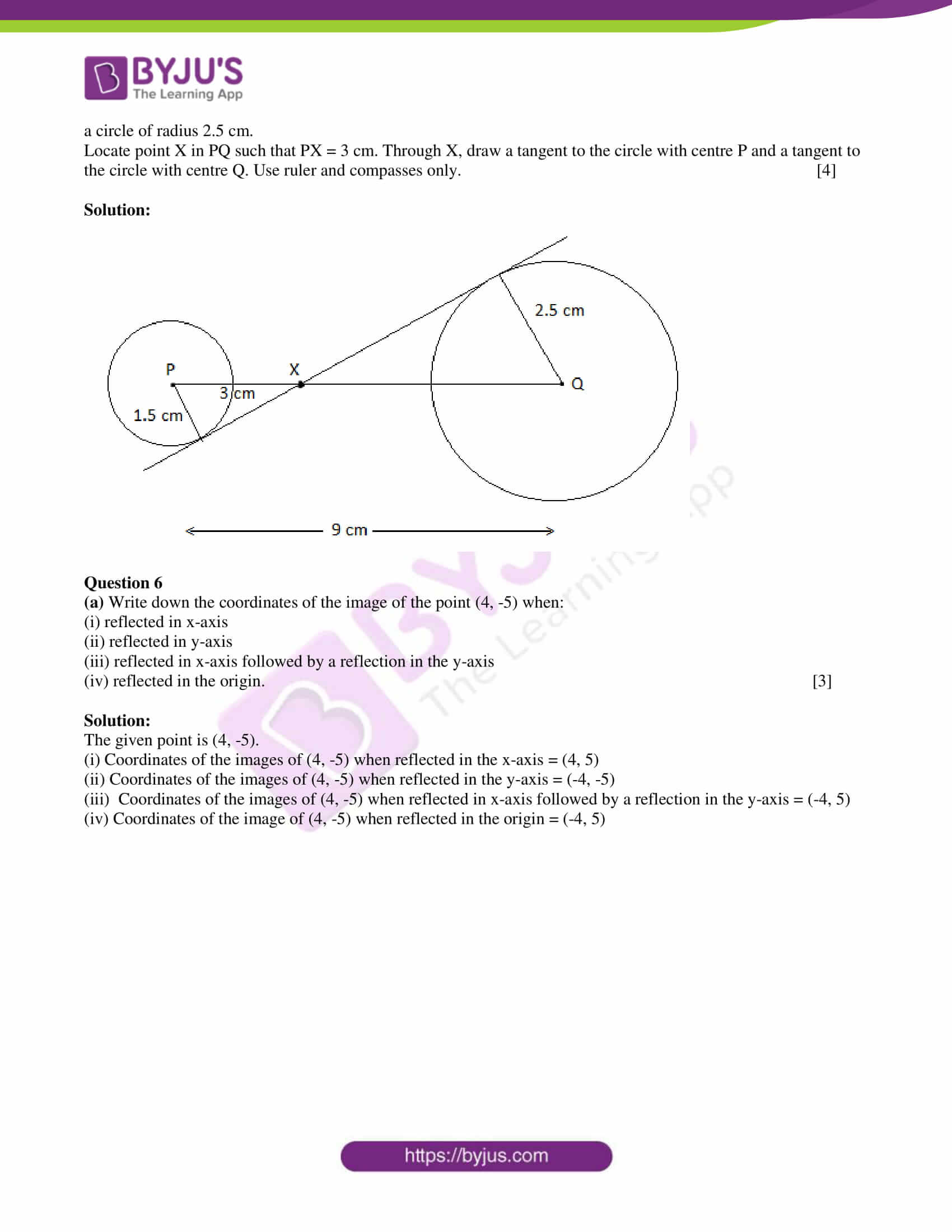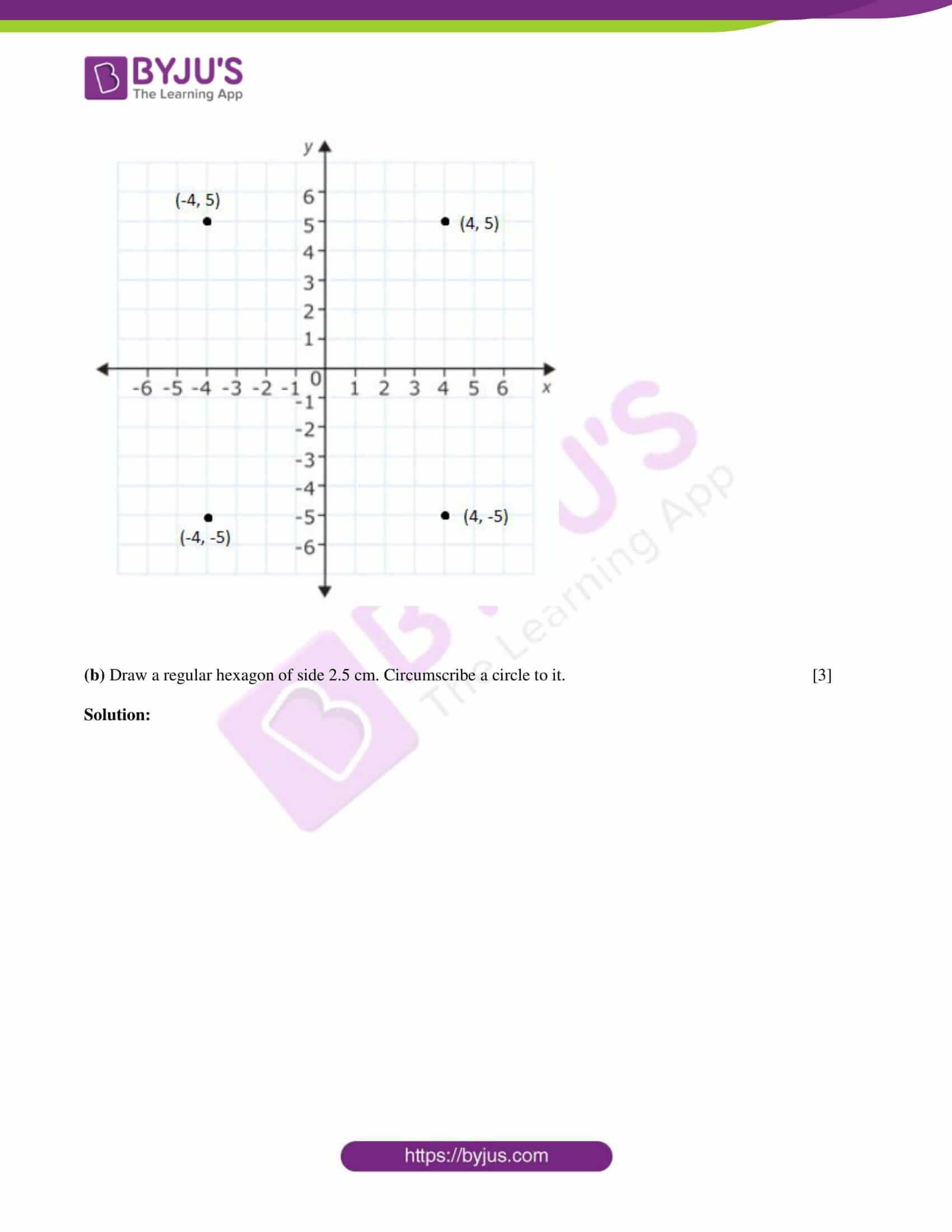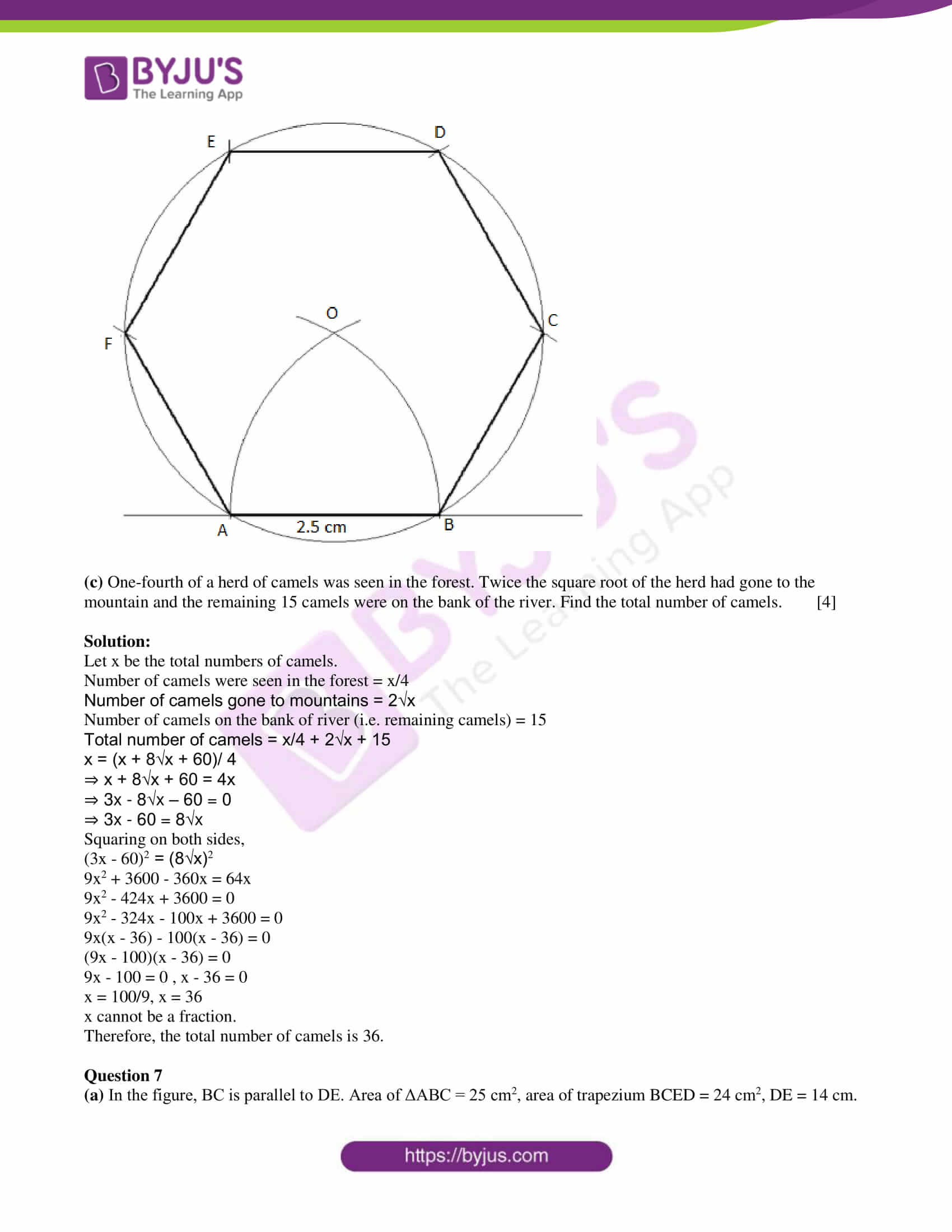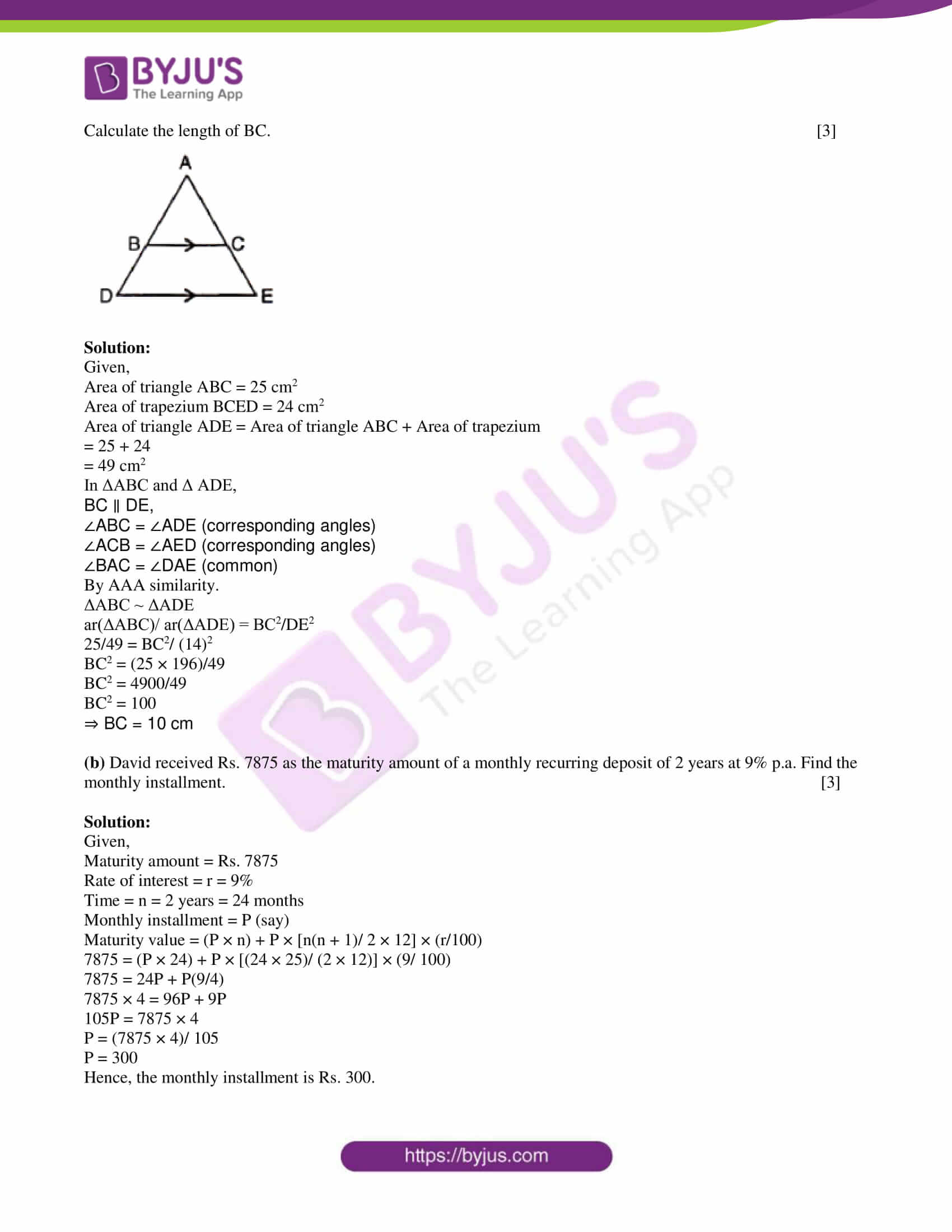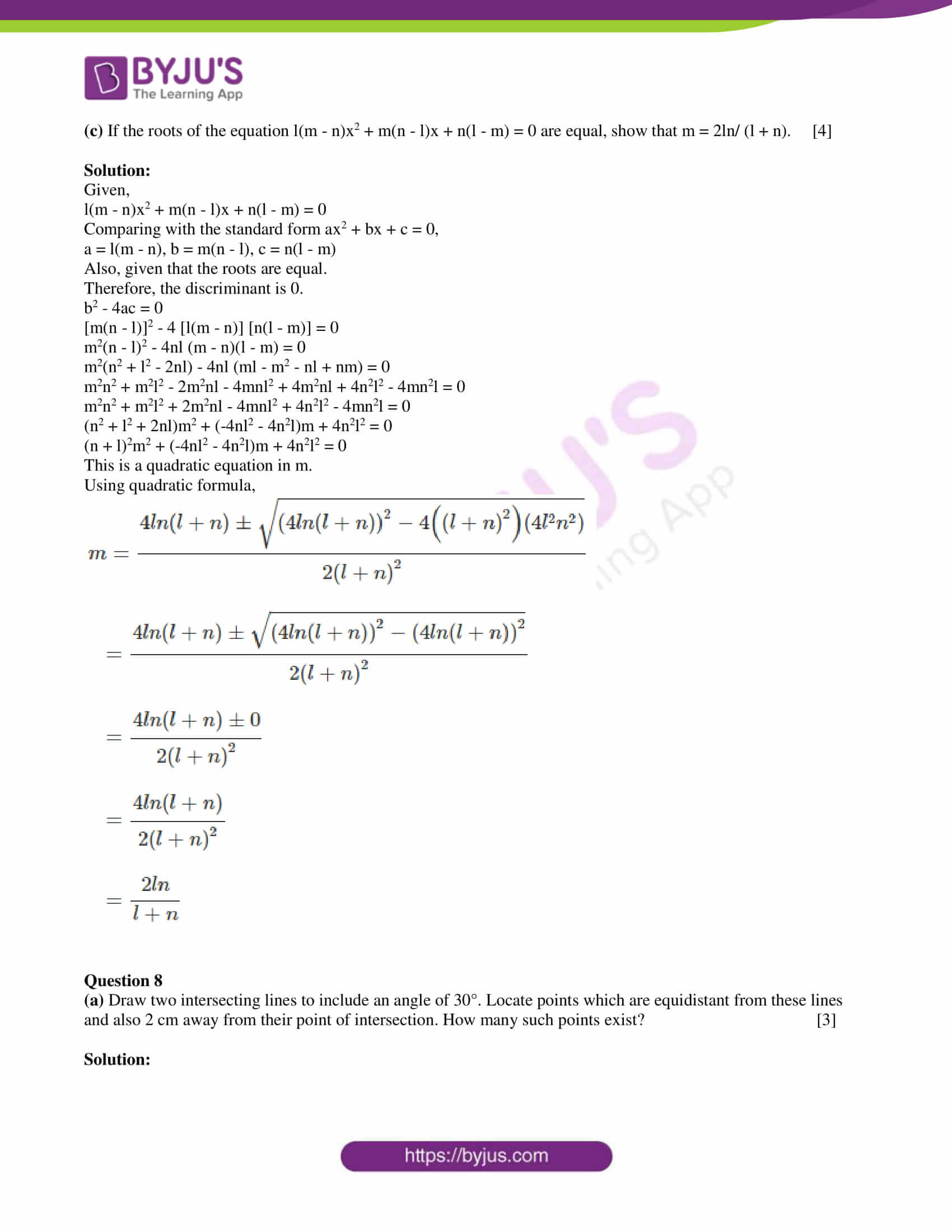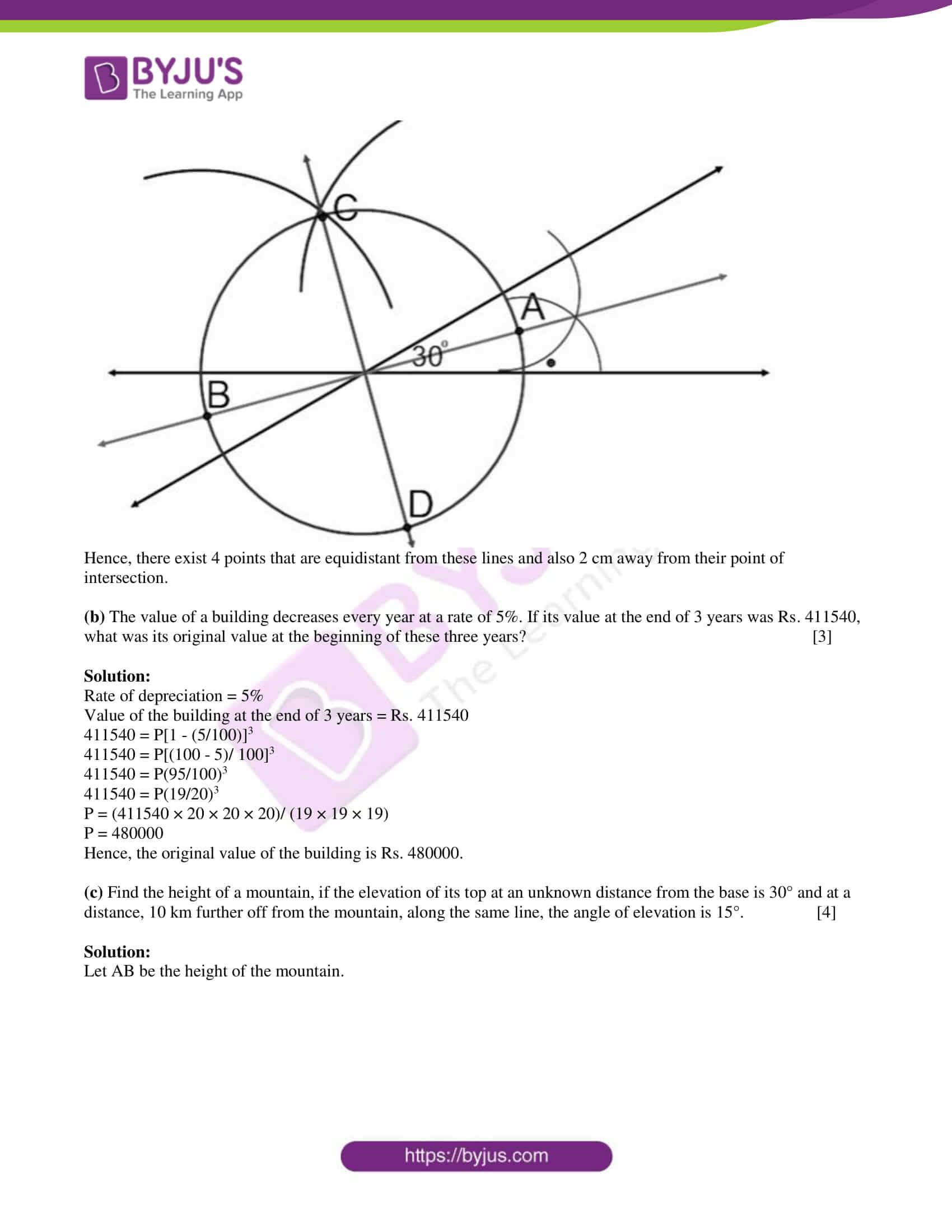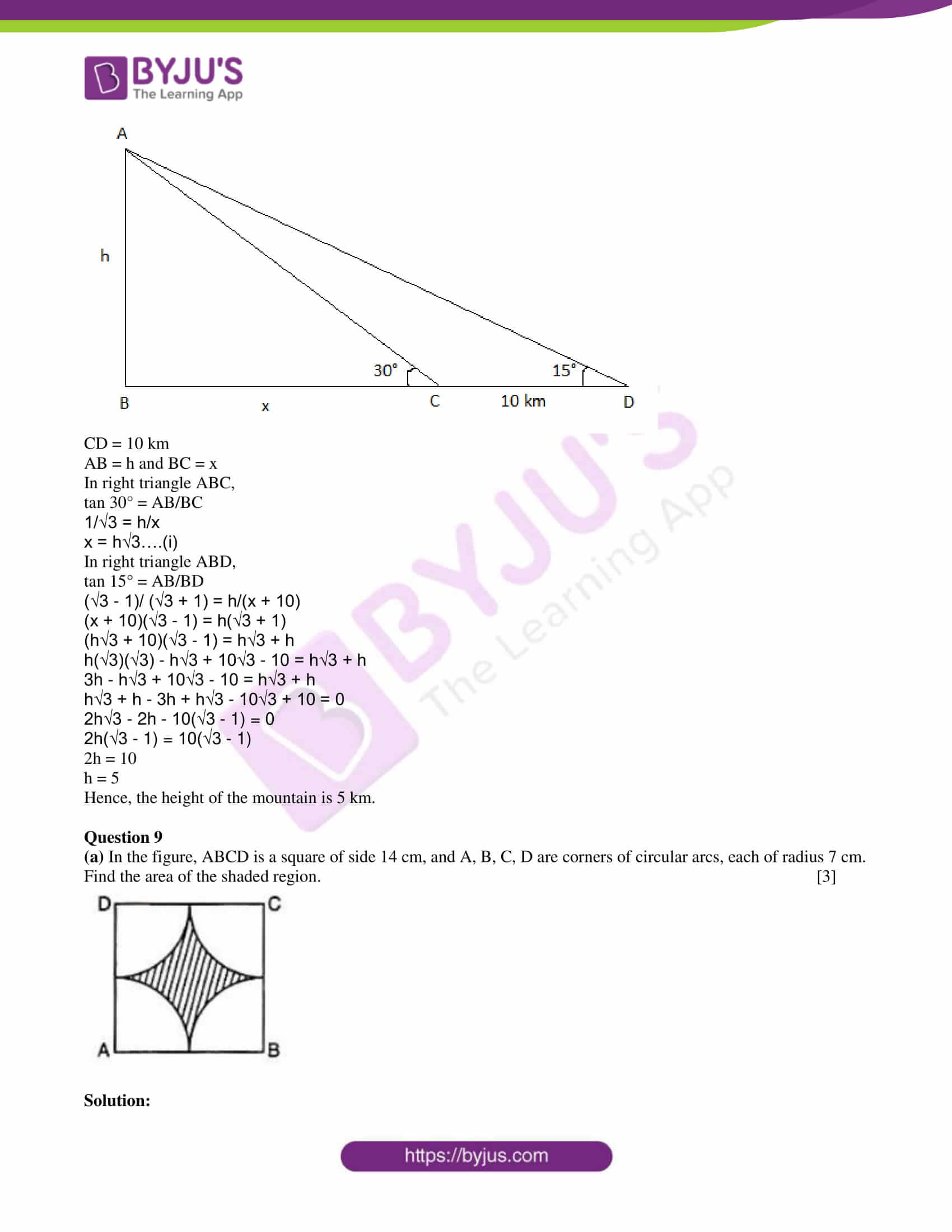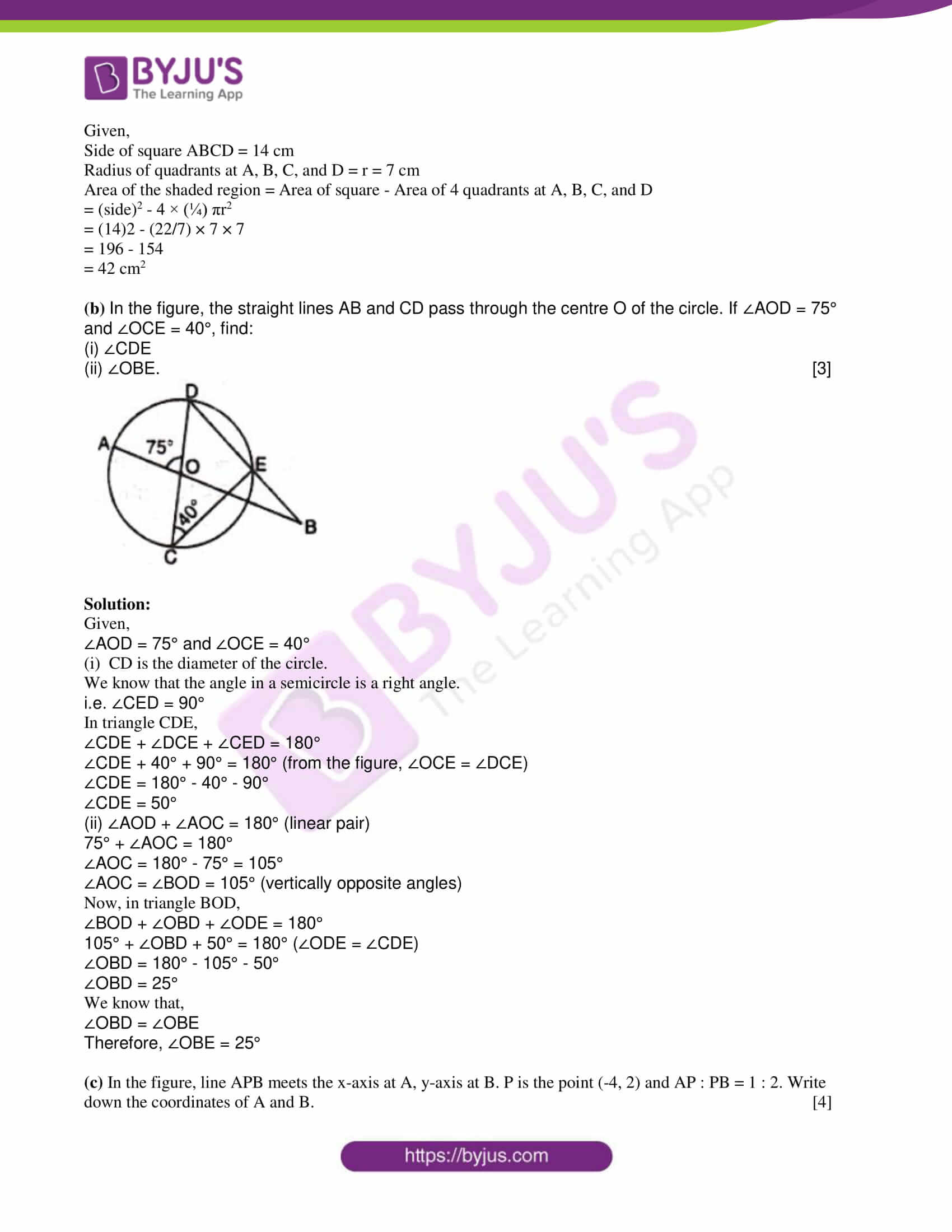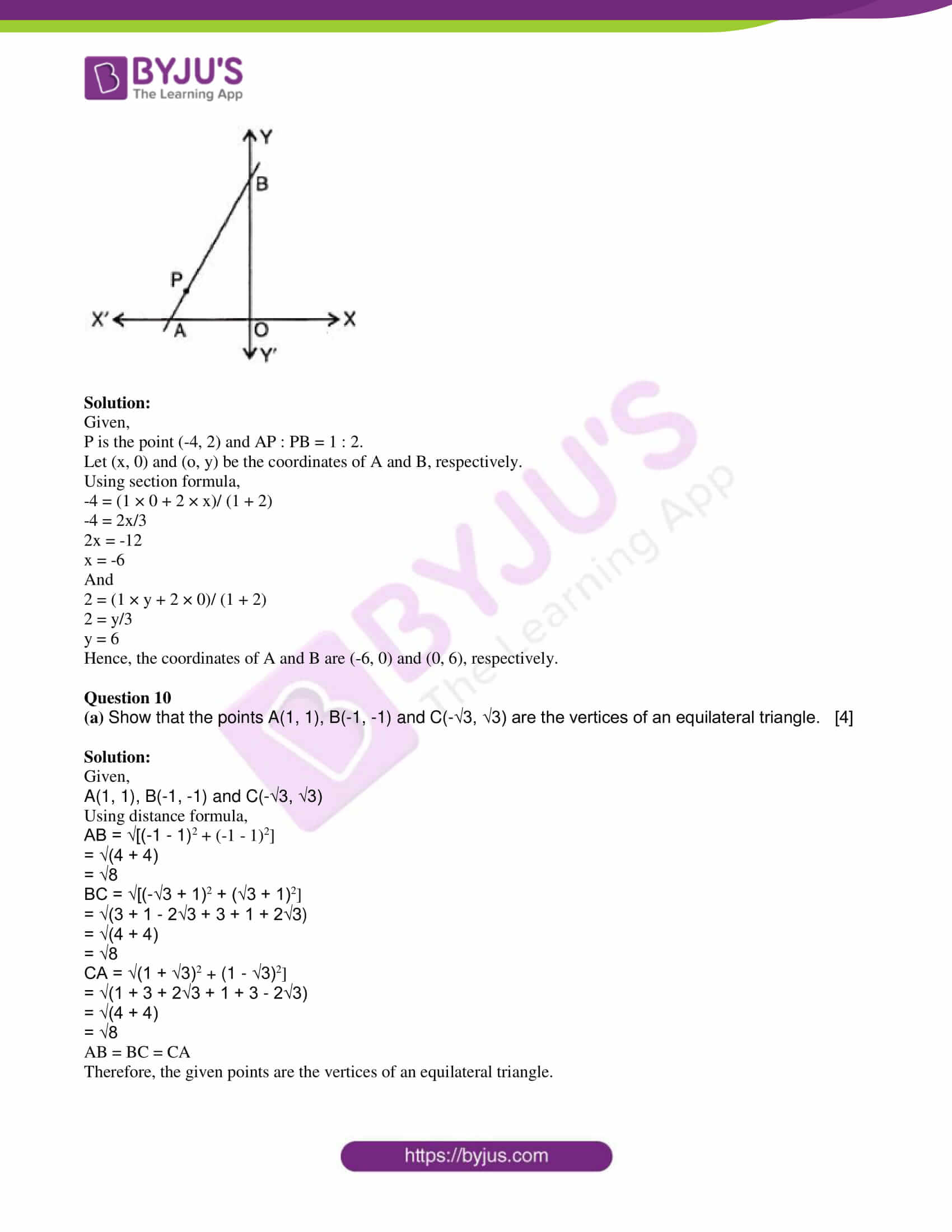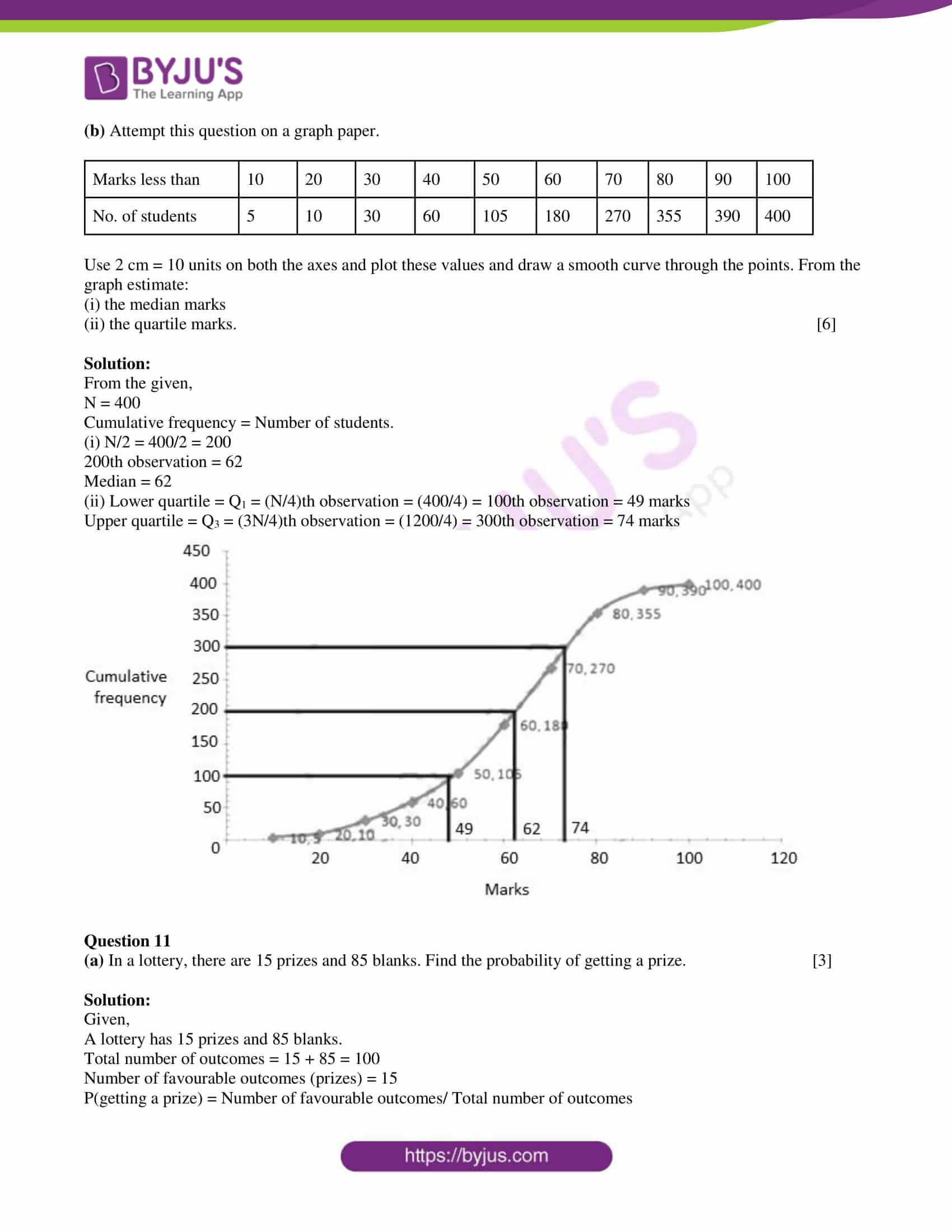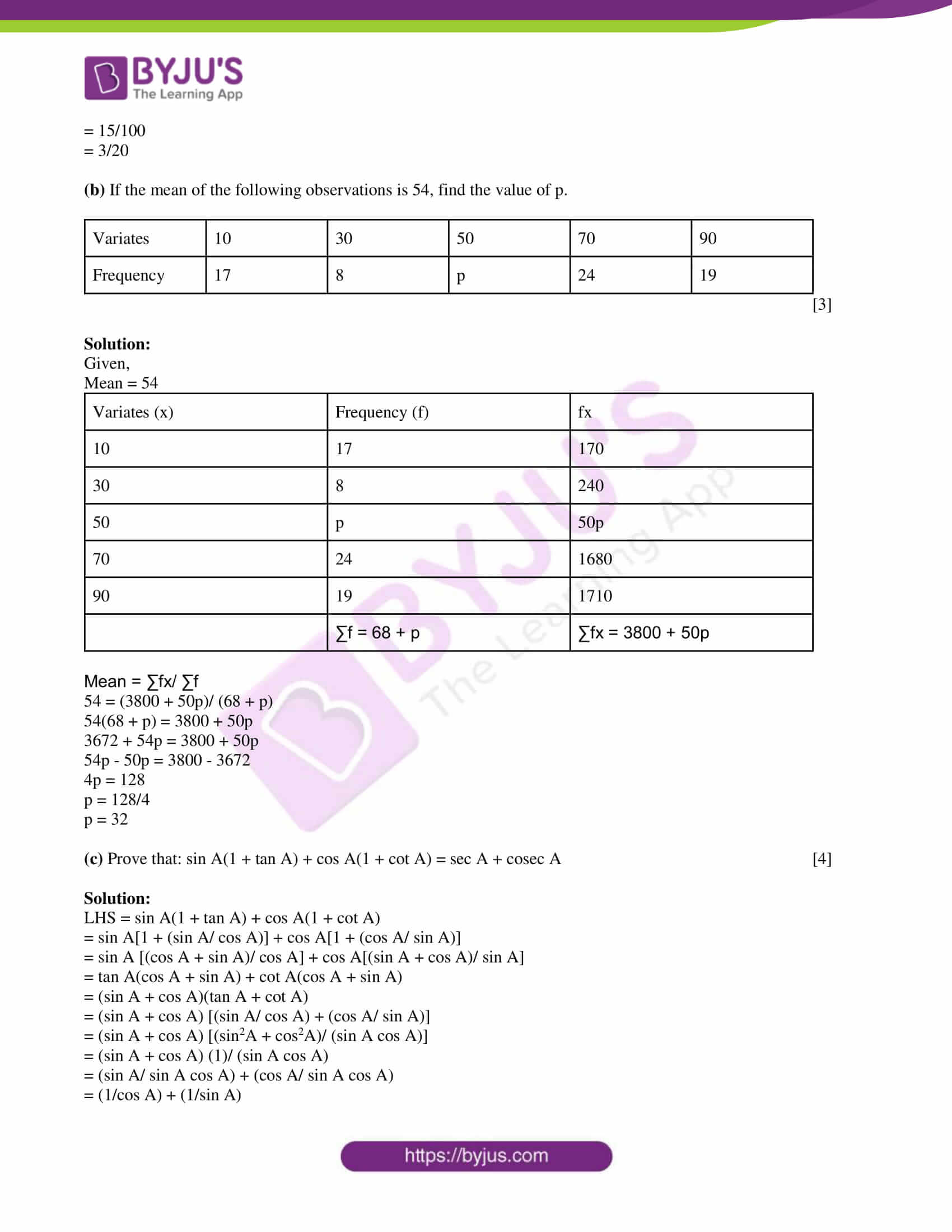SECTION A

Attempt all questions from this section.

Question 1

(a) In the figure, ABCD is a cyclic quadrilateral, O is the centre of the circle. If ∠BOD = 160°, find ∠BPD and ∠BCD.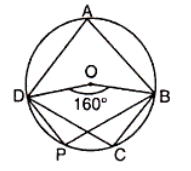Solution:

Given,

∠BOD = 160°

We know that,

= (½) × 160°

= 80°

ABPD is a cyclic quadrilateral. (from the given figure)

The sum of the opposite angles of a cyclic quadrilateral is supplementary.

80° + ∠BPD = 180°

∠BPD = 180° – 80°

∠BPD = 100°

Also, angles in the same segment are equal.

Therefore, ∠BPD = ∠BCD = 100°

(b) Using a ruler and a pair of compasses, construct a rectangle ABCD in which AB = 5 cm and AD = 3 cm. Construct its line of symmetry. 

Solution: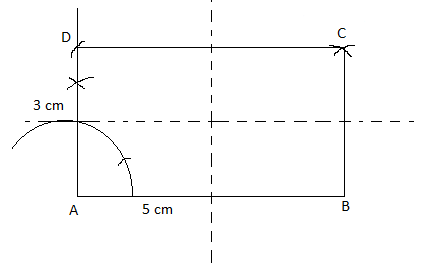(c)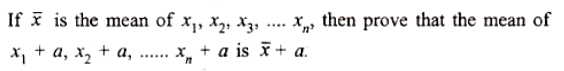Solution:

Given,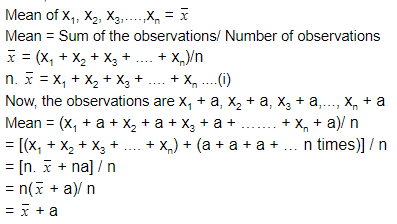Hence proved.

Question 2

(a) Without actual division, find the remainder obtained on dividing (3x2 + 5x – 9) by 3x + 2. 

Solution:

Let p(x) = 3x2 + 5x – 9

Now,

3x + 2 = 0

3x = -2

x = -2/3

Substituting x = -⅔ in p(x),

p(-⅔) = 3(-⅔)2 + 5(-⅔) – 9

= 3(4/9) – (10/3) – 9

= (4/3) – (10/3) – 9

= (4 – 10 – 27)/ 3

= -33/3

= -11

Therefore, the remainder is -11.

(b)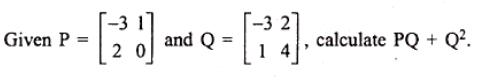Solution:

Given,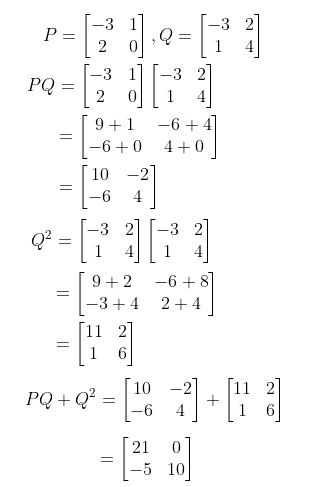(c) Find the range of values of x, which satisfy -⅓ < x/2 – 1 ⅓ < ⅙. 

Solution:

-⅓ < x/2 – 1 ⅓ < ⅙

-⅓ < x/2 – 4/3 < ⅙

(-⅓) + (4/3) < x/2 < (⅙) + (4/3)

3/3 < x/2 < 9/6

1 < x/2 < 3/2

2(1) < x < 2(3/2)

2 < x < 3

Therefore, the range of values of x is 2 < x < 3.

Question 3

(a) If (3a + 5b) : (3a – 5b) = (3c + 5d) : (3c – 5d), show that a : b = c : d 

Solution:

Given,

(3a + 5b) : (3a – 5b) = (3c + 5d) : (3c – 5d)

i.e (3a + 5b)/ (3a – 5b) = (3c + 5d)/ (3c – 5d)

Using componendo and dividendo rule,

(3a + 5b + 3a – 5b)/ (3a + 5b – 3a + 5b) = (3c + 5d + 3c – 5d)/ (3c + 5d – 3c + 5d)

6a/ 10b = 6c/10d

⇒ a/b = c/d

Therefore, a : b = c : d

(b) A spherical ball of iron has been melted and recast into smaller ones. If the radius of each of the smaller balls is one-fourth of the original one, how many such balls can be made? How does the total surface area of the smaller balls compare with that of the original one? 

Solution:

Let R be the radius of the original ball and r be the radius of smaller spherical balls.

Given,

r = R/4

⇒ R = 4r

The volume of original ball = (4/3)πR3

= (4/3)π(4r)3

The volume of smaller ball = (4/3)πr3

Number of balls = Volume of original ball/ Volume of one small ball

= [(4/3)π(4r)3] / [(4/3)πr3]

= 64r3/ r3

= 64

Now, S1 be the surface area of the original spherical ball and S2 be the volume of 64 smaller balls.

S1/S2 = (4πR2)/ (64 × 4πr2)

= [4π(4r)2]/ [64 × 4πr2]

= 16/64

S1/S2 = 1/4

⇒ S2 = 4S1

Therefore, the surface area of smaller balls is four times the surface area of the original ball.

(c) Entries in a savings account passbook are as follows:

 Date Particulars Withdrawn (Rs) Deposited (Rs) Balance (Rs) 4-2-2003 B/F _ _ 2150 10-2-2003 By cash _ 350 2500 1-5-2003 To cheque 400 _ 2100 21-5-2003 By cash _ 400 2500 2-7-2003 To cheque 1500 _ 1000

Calculate the interest for the six months (February to July) at 4.5% p.a. on minimum balance on or after the 10th day of each month. 

Solution:

 Month Minimum balance on or after 10th day (i.e. between 10th and the last day of the month) Feb 2500 March 2500 Apr 2500 May 2100 June 2500 July 1000

Total = Rs. 13100

Rate of interest = R = 4.5%

Time = 6 months

I = PTR/100

= (13100 × 4.5 × 6)/ (12 × 100)

= 294.75

Hence, the interest for six months is Rs. 294.75.

Question 4

(a) Solve for θ: 2 cos 3θ = 1, 0° < θ < 90°. 

Solution:

Given,

2 cos 3θ = 1

cos 3θ = ½

cos 3θ = cos π/3 or cos 3θ = cos (5π/3)

cos 3θ = cos 60° or cos 3θ = cos 300°

3θ = 60° or 3θ = 300°

θ = 20° or θ = 100°

θ = 100° is not possible since 0° < θ < 90°.

Therefore, θ = 20°

(b) Find the unknown length x in the figure.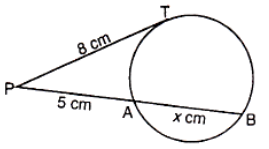Solution:

From the given,

PT = tangent

PAB = secant

We know that,

PT2 = PA × PB

(8)2 = 5 × (PA + AB)

64/5 = (5 + x)

x = (64/5) – 5

x = (64 – 25)/5

x = 39/5

x = 7.8 cm

(c) An article is available for Rs. 6048 inclusive of sales tax at the rate of 8%. Find its list price. What will be its new selling price if the rate of sales tax changes to 12%? 

Solution:

Given,

Price of an article inclusive of sales tax = Rs. 6048

Sales tax = 8%

List price = (100/ 108) × Rs. 6048

= Rs. 5600

New sales tax = 12%

Selling price = (112/100) × Rs. 5600

= Rs. 6272

Therefore, the new selling price of the article is Rs. 6272.

SECTION – B

Attempt any four questions from this section.

Question 5

(a) A part of Rs. 3020 is invested in 6% Rs. 100 shares at Rs. 97 and the rest in 12% Rs. 100 shares at Rs. 108. If both bring the same dividend, find:

(i) the sum invested in the shares selling at a discount

(ii) the sum invested in the shares selling above par

(iii) total dividend. 

Solution:

Let x be the number of shares invested at 6% and y be the number of shares invested at 12%.

According to the given,

97x + 108y = 3020…..(i)

Also, the dividend is the same in both cases.

6x = 12y

x = 2y….(ii)

From (i) and (ii),

97(2y) + 108y = 3020

194y + 108y = 3020

302y = 3020

y = 3020/ 302

y = 10

Therefore, x = 20

(i) Amount invested in 6% = 97x = 97 × 20 = Rs. 1940

Thus, the sum invested in the shares selling at a discount is Rs. 1940.

(ii) Amount invested in 12% = 108y = 108 × 10 = Rs. 1080

Thus, the sum invested in the shares selling above par is Rs. 1080.

(iii) Total dividend = 6x + 12y

= 6(20) + 12(10)

= 120 + 120

= Rs. 240

(b)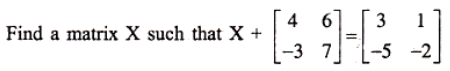Solution:

Given,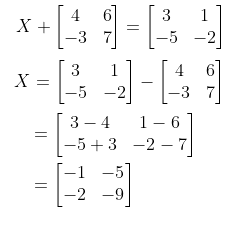(c) Take points P and Q at a distance of 9 cm from each other. At P, draw a circle of radius 1.5 cm and at Q, draw a circle of radius 2.5 cm.

Locate point X in PQ such that PX = 3 cm. Through X, draw a tangent to the circle with centre P and a tangent to the circle with centre Q. Use ruler and compasses only. 

Solution: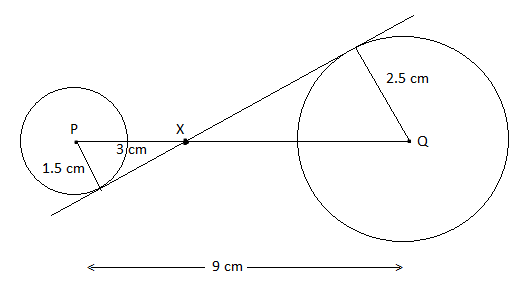Question 6

(a) Write down the coordinates of the image of the point (4, -5) when:

(i) reflected in x-axis

(ii) reflected in y-axis

(iii) reflected in x-axis followed by a reflection in the y-axis

(iv) reflected in the origin. 

Solution:

The given point is (4, -5).

(i) Coordinates of the images of (4, -5) when reflected in the x-axis = (4, 5)

(ii) Coordinates of the images of (4, -5) when reflected in the y-axis = (-4, -5)

(iii) Coordinates of the images of (4, -5) when reflected in x-axis followed by a reflection in the y-axis = (-4, 5)

(iv) Coordinates of the image of (4, -5) when reflected in the origin = (-4, 5)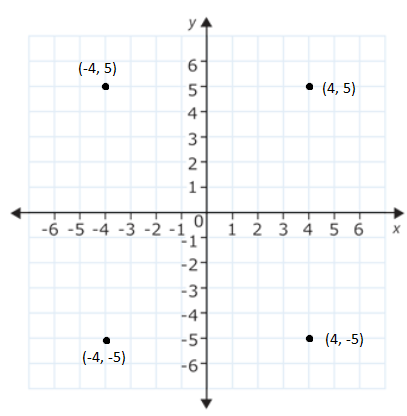(b) Draw a regular hexagon of side 2.5 cm. Circumscribe a circle to it. 

Solution: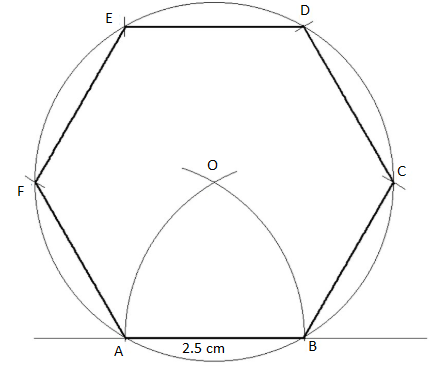(c) One-fourth of a herd of camels was seen in the forest. Twice the square root of the herd had gone to the mountain and the remaining 15 camels were on the bank of the river. Find the total number of camels. 

Solution:

Let x be the total numbers of camels.

Number of camels were seen in the forest = x/4

Number of camels gone to mountains = 2√x

Number of camels on the bank of river (i.e. remaining camels) = 15

Total number of camels = x/4 + 2√x + 15

x = (x + 8√x + 60)/ 4

⇒ x + 8√x + 60 = 4x

⇒ 3x – 8√x – 60 = 0

⇒ 3x – 60 = 8√x

Squaring on both sides,

(3x – 60)2 = (8√x)2

9x2 + 3600 – 360x = 64x

9x2 – 424x + 3600 = 0

9x2 – 324x – 100x + 3600 = 0

9x(x – 36) – 100(x – 36) = 0

(9x – 100)(x – 36) = 0

9x – 100 = 0 , x – 36 = 0

x = 100/9, x = 36

x cannot be a fraction.

Therefore, the total number of camels is 36.

Question 7

(a) In the figure, BC is parallel to DE. Area of ΔABC = 25 cm2, area of trapezium BCED = 24 cm2, DE = 14 cm. Calculate the length of BC.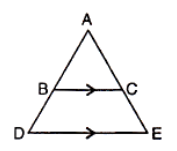Solution:

Given,

Area of triangle ABC = 25 cm2

Area of trapezium BCED = 24 cm2

Area of triangle ADE = Area of triangle ABC + Area of trapezium

= 25 + 24

= 49 cm2

BC ∥ DE,

∠ACB = ∠AED (corresponding angles)

∠BAC = ∠DAE (common)

By AAA similarity.

25/49 = BC2/ (14)2

BC2 = (25 × 196)/49

BC2 = 4900/49

BC2 = 100

⇒ BC = 10 cm

(b) David received Rs. 7875 as the maturity amount of a monthly recurring deposit of 2 years at 9% p.a. Find the monthly installment. 

Solution:

Given,

Maturity amount = Rs. 7875

Rate of interest = r = 9%

Time = n = 2 years = 24 months

Monthly installment = P (say)

Maturity value = (P × n) + P × [n(n + 1)/ 2 × 12] × (r/100)

7875 = (P × 24) + P × [(24 × 25)/ (2 × 12)] × (9/ 100)

7875 = 24P + P(9/4)

7875 × 4 = 96P + 9P

105P = 7875 × 4

P = (7875 × 4)/ 105

P = 300

Hence, the monthly installment is Rs. 300.

(c) If the roots of the equation l(m – n)x2 + m(n – l)x + n(l – m) = 0 are equal, show that m = 2ln/ (l + n). 

Solution:

Given,

l(m – n)x2 + m(n – l)x + n(l – m) = 0

Comparing with the standard form ax2 + bx + c = 0,

a = l(m – n), b = m(n – l), c = n(l – m)

Also, given that the roots are equal.

Therefore, the discriminant is 0.

b2 – 4ac = 0

[m(n – l)]2 – 4 [l(m – n)] [n(l – m)] = 0

m2(n – l)2 – 4nl (m – n)(l – m) = 0

m2(n2 + l2 – 2nl) – 4nl (ml – m2 – nl + nm) = 0

m2n2 + m2l2 – 2m2nl – 4mnl2 + 4m2nl + 4n2l2 – 4mn2l = 0

m2n2 + m2l2 + 2m2nl – 4mnl2 + 4n2l2 – 4mn2l = 0

(n2 + l2 + 2nl)m2 + (-4nl2 – 4n2l)m + 4n2l2 = 0

(n + l)2m2 + (-4nl2 – 4n2l)m + 4n2l2 = 0

This is a quadratic equation in m.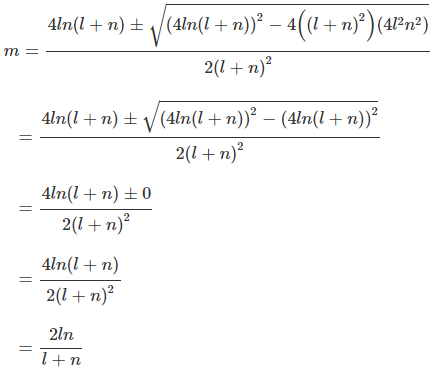Question 8

(a) Draw two intersecting lines to include an angle of 30°. Locate points which are equidistant from these lines and also 2 cm away from their point of intersection. How many such points exist? 

Solution: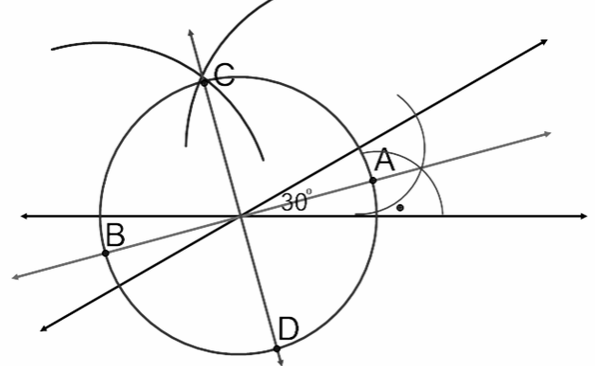Hence, there exist 4 points that are equidistant from these lines and also 2 cm away from their point of intersection.

(b) The value of a building decreases every year at a rate of 5%. If its value at the end of 3 years was Rs. 411540, what was its original value at the beginning of these three years? 

Solution:

Rate of depreciation = 5%

Value of the building at the end of 3 years = Rs. 411540

411540 = P[1 – (5/100)]3

411540 = P[(100 – 5)/ 100]3

411540 = P(95/100)3

411540 = P(19/20)3

P = (411540 × 20 × 20 × 20)/ (19 × 19 × 19)

P = 480000

Hence, the original value of the building is Rs. 480000.

(c) Find the height of a mountain, if the elevation of its top at an unknown distance from the base is 30° and at a distance, 10 km further off from the mountain, along the same line, the angle of elevation is 15°. 

Solution:

Let AB be the height of the mountain.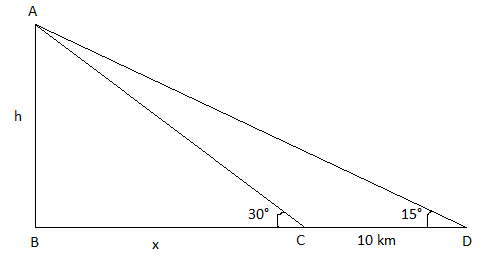CD = 10 km

AB = h and BC = x

In right triangle ABC,

tan 30° = AB/BC

1/√3 = h/x

x = h√3….(i)

In right triangle ABD,

tan 15° = AB/BD

(√3 – 1)/ (√3 + 1) = h/(x + 10)

(x + 10)(√3 – 1) = h(√3 + 1)

(h√3 + 10)(√3 – 1) = h√3 + h

h(√3)(√3) – h√3 + 10√3 – 10 = h√3 + h

3h – h√3 + 10√3 – 10 = h√3 + h

h√3 + h – 3h + h√3 – 10√3 + 10 = 0

2h√3 – 2h – 10(√3 – 1) = 0

2h(√3 – 1) = 10(√3 – 1)

2h = 10

h = 5

Hence, the height of the mountain is 5 km.

Question 9

(a) In the figure, ABCD is a square of side 14 cm, and A, B, C, D are corners of circular arcs, each of radius 7 cm. Find the area of the shaded region.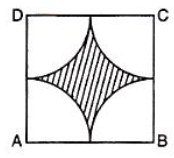Solution:

Given,

Side of square ABCD = 14 cm

Radius of quadrants at A, B, C, and D = r = 7 cm

Area of the shaded region = Area of square – Area of 4 quadrants at A, B, C, and D

= (side)2 – 4 × (¼) πr2

= (14)2 – (22/7) × 7 × 7

= 196 – 154

= 42 cm2

(b) In the figure, the straight lines AB and CD pass through the centre O of the circle. If ∠AOD = 75° and ∠OCE = 40°, find:

(i) ∠CDE

(ii) ∠OBE.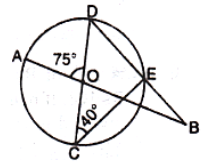Solution:

Given,

∠AOD = 75° and ∠OCE = 40°

(i) CD is the diameter of the circle.

We know that the angle in a semicircle is a right angle.

i.e. ∠CED = 90°

In triangle CDE,

∠CDE + ∠DCE + ∠CED = 180°

∠CDE + 40° + 90° = 180° (from the figure, ∠OCE = ∠DCE)

∠CDE = 180° – 40° – 90°

∠CDE = 50°

(ii) ∠AOD + ∠AOC = 180° (linear pair)

75° + ∠AOC = 180°

∠AOC = 180° – 75° = 105°

∠AOC = ∠BOD = 105° (vertically opposite angles)

Now, in triangle BOD,

∠BOD + ∠OBD + ∠ODE = 180°

105° + ∠OBD + 50° = 180° (∠ODE = ∠CDE)

∠OBD = 180° – 105° – 50°

∠OBD = 25°

We know that,

∠OBD = ∠OBE

Therefore, ∠OBE = 25°

(c) In the figure, line APB meets the x-axis at A, y-axis at B. P is the point (-4, 2) and AP : PB = 1 : 2. Write down the coordinates of A and B.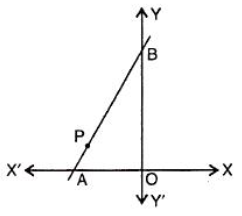Solution:

Given,

P is the point (-4, 2) and AP : PB = 1 : 2.

Let (x, 0) and (o, y) be the coordinates of A and B, respectively.

Using section formula,

-4 = (1 × 0 + 2 × x)/ (1 + 2)

-4 = 2x/3

2x = -12

x = -6

And

2 = (1 × y + 2 × 0)/ (1 + 2)

2 = y/3

y = 6

Hence, the coordinates of A and B are (-6, 0) and (0, 6), respectively.

Question 10

(a) Show that the points A(1, 1), B(-1, -1) and C(-√3, √3) are the vertices of an equilateral triangle. 

Solution:

Given,

A(1, 1), B(-1, -1) and C(-√3, √3)

Using distance formula,

AB = √[(-1 – 1)2 + (-1 – 1)2]

= √(4 + 4)

= √8

BC = √[(-√3 + 1)2 + (√3 + 1)2]

= √(3 + 1 – 2√3 + 3 + 1 + 2√3)

= √(4 + 4)

= √8

CA = √(1 + √3)2 + (1 – √3)2]

= √(1 + 3 + 2√3 + 1 + 3 – 2√3)

= √(4 + 4)

= √8

AB = BC = CA

Therefore, the given points are the vertices of an equilateral triangle.

(b) Attempt this question on a graph paper.

 Marks less than 10 20 30 40 50 60 70 80 90 100 No. of students 5 10 30 60 105 180 270 355 390 400

Use 2 cm = 10 units on both the axes and plot these values and draw a smooth curve through the points. From the graph estimate:

(i) the median marks

(ii) the quartile marks. 

Solution:

From the given,

N = 400

Cumulative frequency = Number of students.

(i) N/2 = 400/2 = 200

200th observation = 62

Median = 62

(ii) Lower quartile = Q1 = (N/4)th observation = (400/4) = 100th observation = 49 marks

Upper quartile = Q3 = (3N/4)th observation = (1200/4) = 300th observation = 74 marks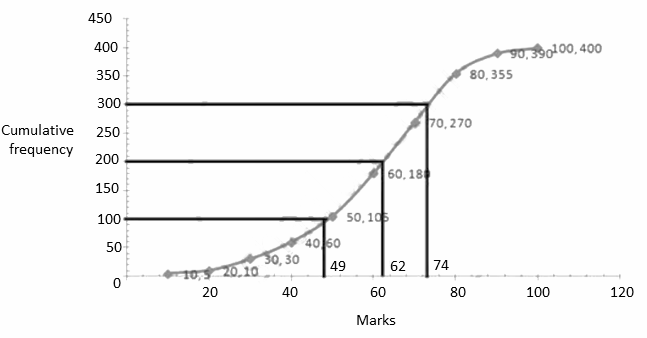Question 11

(a) In a lottery, there are 15 prizes and 85 blanks. Find the probability of getting a prize. 

Solution:

Given,

A lottery has 15 prizes and 85 blanks.

Total number of outcomes = 15 + 85 = 100

Number of favourable outcomes (prizes) = 15

P(getting a prize) = Number of favourable outcomes/ Total number of outcomes

= 15/100

= 3/20

(b) If the mean of the following observations is 54, find the value of p.

 Variates 10 30 50 70 90 Frequency 17 8 p 24 19


Solution:

Given,

Mean = 54

 Variates (x) Frequency (f) fx 10 17 170 30 8 240 50 p 50p 70 24 1680 90 19 1710 ∑f = 68 + p ∑fx = 3800 + 50p

Mean = ∑fx/ ∑f

54 = (3800 + 50p)/ (68 + p)

54(68 + p) = 3800 + 50p

3672 + 54p = 3800 + 50p

54p – 50p = 3800 – 3672

4p = 128

p = 128/4

p = 32

(c) Prove that: sin A(1 + tan A) + cos A(1 + cot A) = sec A + cosec A 

Solution:

LHS = sin A(1 + tan A) + cos A(1 + cot A)

= sin A[1 + (sin A/ cos A)] + cos A[1 + (cos A/ sin A)]

= sin A [(cos A + sin A)/ cos A] + cos A[(sin A + cos A)/ sin A]

= tan A(cos A + sin A) + cot A(cos A + sin A)

= (sin A + cos A)(tan A + cot A)

= (sin A + cos A) [(sin A/ cos A) + (cos A/ sin A)]

= (sin A + cos A) [(sin2A + cos2A)/ (sin A cos A)]

= (sin A + cos A) (1)/ (sin A cos A)

= (sin A/ sin A cos A) + (cos A/ sin A cos A)

= (1/cos A) + (1/sin A)

= sec A + cosec A

= RHS

Hence proved.